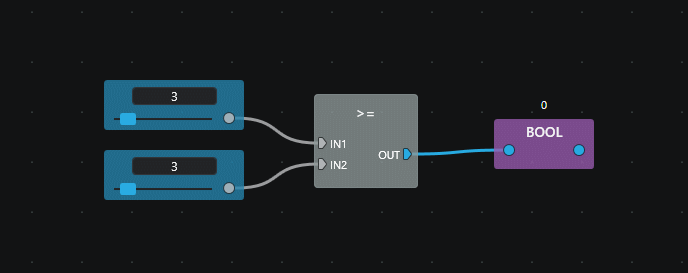# Relational and Equality¶## Less Than (<)¶

Compares if the first operand (IN1) is less than the second (IN2).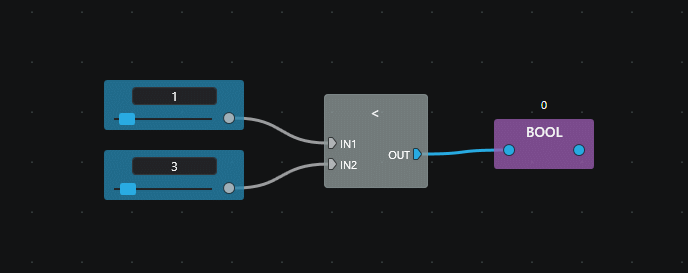## Less or Equal (<=)¶

Compares if the first operand (IN1) is less than or equal to the second (IN2).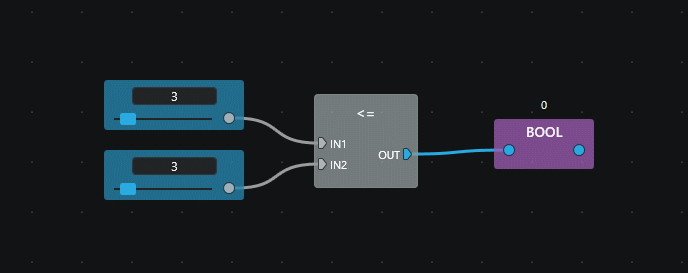## Equal (=)¶

Compares if the first operand (IN1) is equal to the second (IN2).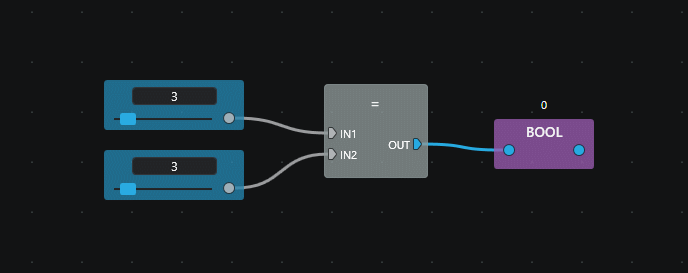## Not Equal (≠)¶

Compares if the first operand (IN1) is different than the second (IN2).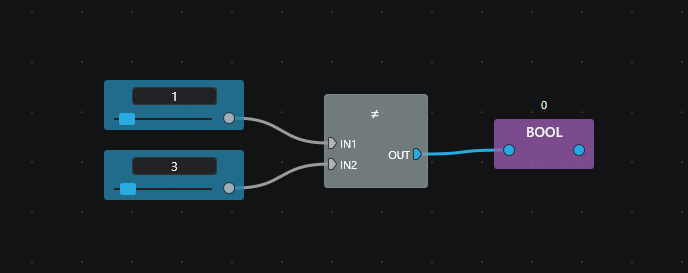## Greater Than (>)¶

Compares if the first operand (IN1) is greater than the second (IN2).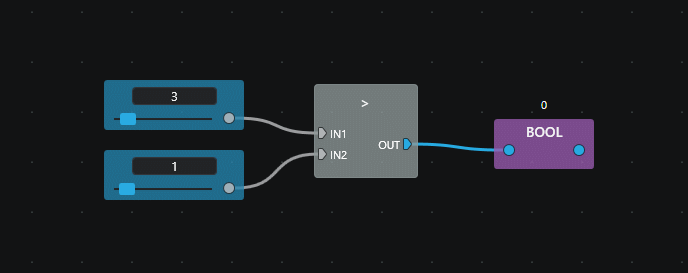## Greater or Equal (>=)¶

Compares if te first operand (IN1) is greater than or equal to the second (IN2).